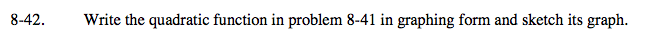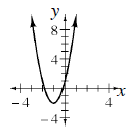### Home > A2C > Chapter 8 > Lesson 8.1.3 > Problem8-42

8-42.Factor out the 3 and separate it from the constant.

Complete the square.
Add the value that completes the square inside the parentheses
and subtract it outside.
(Why is the value subtracted 3 not 1?)

y = 3x2 + 6x + 1
y = 3(x2 + 2x) + 1

y = 3(x2 + 2x + 1) + 1 − 3

y = 3(x + 1)2 − 2